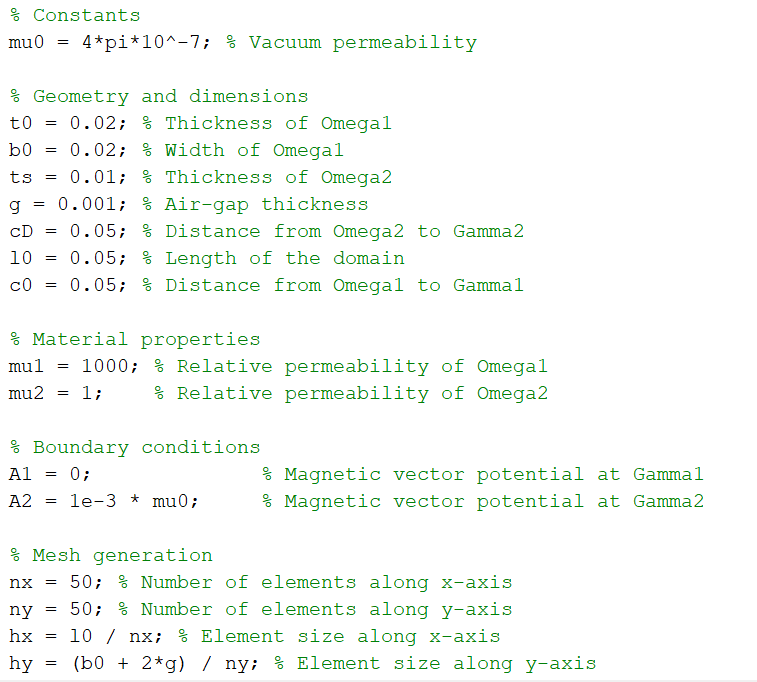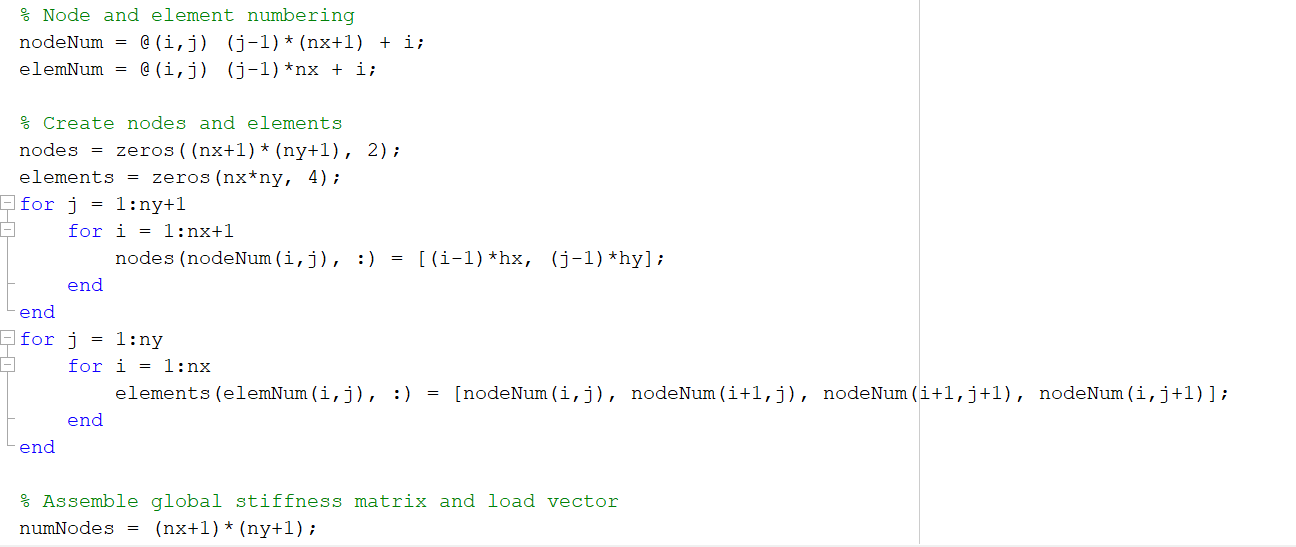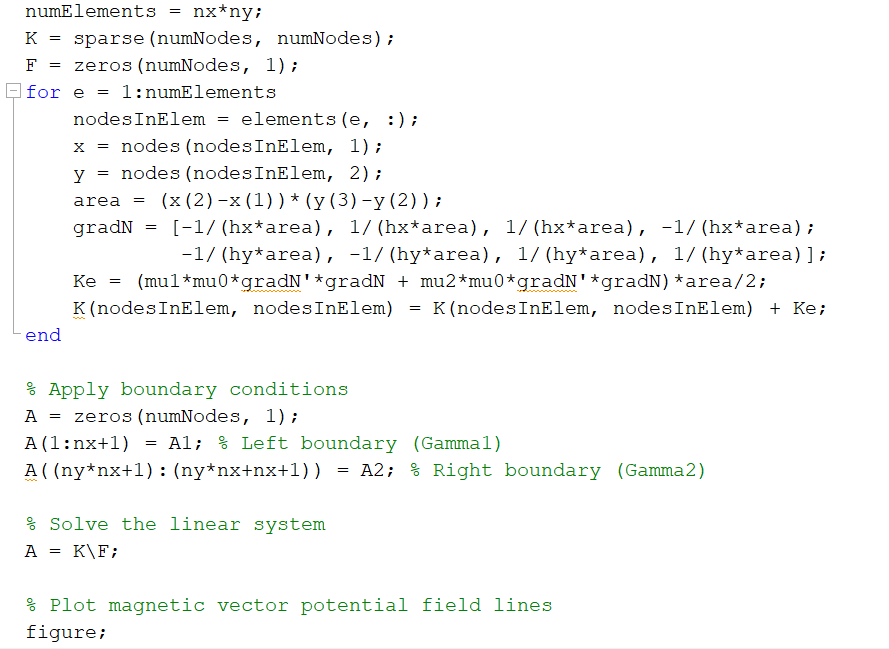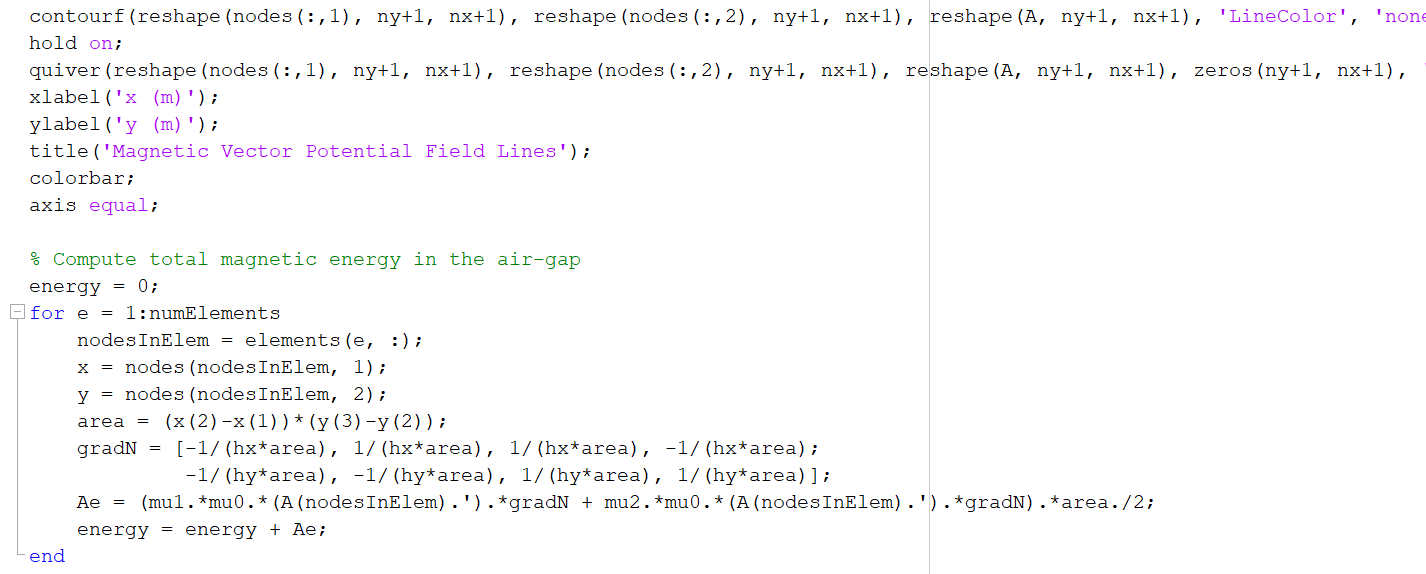Home / Expert Answers / Electrical Engineering / trying-to-figure-this-error-error-using-horzcat-dimensions-of-arrays-being-concatenated-are-not-co-pa349

# (Solved): trying to figure this error: Error using horzcat Dimensions of arrays being concatenated are not co ...trying to figure this error:

Error using horzcat
Dimensions of arrays being concatenated are not consistent.

Constants mu0 ㅇacuum permeability \% Geometry and dimensions t0 Thickness of Omegal Width of Omegal Thickness of Omega2 Air-gap thickness Distance from Omega2 to Gamma2 Length of the domain Distance from Omegal to Gammal \% Material properties mu1 o Relative permeability of Omegal mu2 Relative permeability of Omega 2 Boundary conditions o Magnetic vector potential at Gammal A2 응 Magnetic vector potential at Gamma2 Mesh generation Number of elements along -axis Number of elements along -axis Element size along -axis ny; Element size along -axis \% Node and element numbering nodeNum elemNum i \% Create nodes and elements nodes ; elements ; for for nodes end -end for for elements (elemNum end -end \% Assemble global stiffness matrix and load vector numNodes numElements sparse (numNodes, numNodes); (numNodes, 1); for :numElements nodesInElem = elements ; nodes (nodesInElem, 1 ); nodes (nodesInElem, 2); area * ; *area ; (nodesInElem, nodesInElem) (nodesInElem, nodesInElem) ; end \% Apply boundary conditions (numNodes, 1); Left boundary (Gamma1) Right boundary (Gamma2) ° Solve the linear system ; \% Plot magnetic vector potential field lines figure; contourf(reshape(nodes , ny+1, , reshape(nodes , reshape(A, ny+1, nx+1), 'LineColor', 'non hold on: quiver (reshape (nodes , , reshape (nodes , reshape (A, , , zeros (ny+1, , title('Magnetic Vector Potential Field Lines'); colorbar; axis equal; ° Compute total magnetic energy in the air-gap energy ; for numElements nodesInElem = elements ; nodesInElem, 1 ); nodes (nodesInElem, 2); area ; energy = energy + Ae; end

We have an Answer from Expert

The error message "Error using  horzcat "  : Dimensions of arrays being concatenated are not consistent" occurs when you are trying to concatenate arrays horizontally (using the horzcat function or the